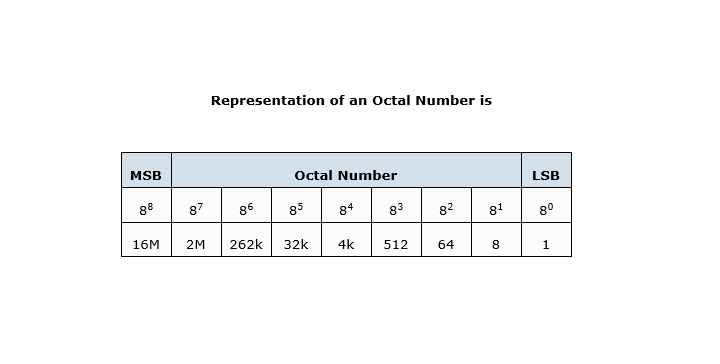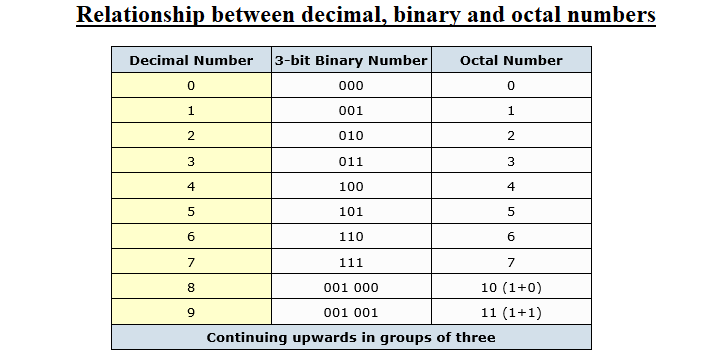# Octal Number System | Octal to Decimal Binary & Hexadecimal Conversion

The Octal Number System is another type of computer and digital base number system.

Octal Number System is very similar in principle to the previous hexadecimal numbering system except that in Octal, a binary number is divided up into groups of only 3 bits, with each group or set of bits having a distinct value of between 000 (0) and 111 ( 4+2+1 = 7 ).

The octal numbers therefore have a range of just “8” digits, (0, 1, 2, 3, 4, 5, 6, 7) making them a Base-8 numbering system and therefore, q is equal to “8”.

Then the main characteristics of an Octal Number System is that there are only 8 distinct counting digits from 0 to 7 with each digit having a weight or value of just 8 starting from the least significant bit (LSB).

In the earlier days of computing, octal numbers and the octal numbering system was very popular for counting inputs and outputs because as it works in counts of eight, inputs and outputs were in counts of eight, a byte at a time.

As the base of an octal number system is 8 (base-8), which also represents the number of individual numbers used in the system, the subscript 8 is used to identify a number expressed in octal.

For example, an octal number is expressed as: 2378

Just like the hexadecimal system, the “octal number system” provides a convenient way of converting large binary numbers into more compact and smaller groups.

However, these days the octal numbering system is used less frequently than the hexadecimal number system.As the octal number system uses only eight digits (0 through 7) there are no numbers or letters used above 8, but the conversion from decimal to octal and binary to octal follows the same pattern as we have seen previously for hexadecimal.

To count above 7 in octal we need to add another column and start over again in a similar way to hexadecimal. 0, 1, 2, 3, 4, 5, 6, 7, 10, 11, 12, 13, 14, 15, 16, 17, 20, 21… etc.

Again do not get confused, 10 or 20 is NOT ten or twenty it is 1 + 0 and 2 + 0 in octal exactly the same as for hexadecimal.

## Binary to Octal Representation

The relationship between decimal binary and octal numbers is given below.Then we can see that 1 octal number or digit is equivalent to 3 bits, and with two octal number, 778 we can count up to 63 in decimal, with three octal numbers, 7778 up to 511 in decimal and with four octal numbers, 77778 up to 4095 in decimal and so on.

Octal Numbers Example No 1

Using our previous binary number of 11010101110011112 convert this binary number to its octal equivalent, (base-2 to base-8).

Binary Digit Value 001101010111001111

Group the bits into three´s starting from the right hand side 001 101 010 111 001 111

Octal Number form 1527178

Thus, 0011010101110011112 in its Binary form is equivalent to 1527178 in Octal form or 54,735 in denary.

### octal to decimal example No 2

Convert the octal number 23228 to its decimal number equivalent, (base-8 to base-10).

Octal Digit Value 23228

In polynomial form = ( 2×83 ) + ( 3×82 ) + ( 2×81 ) + ( 2×80 )

Add the results = ( 1024 ) + ( 192 ) + ( 16 ) + ( 2 )

Decimal number form equals: 123410

Then, converting octal to decimal shows that 23228 in its Octal form is equivalent to 123410 in its Decimal form.

While Octal is another type of digital numbering system, it is little used these days instead the more commonly Hexadecimal Numbering System is used as it is more flexible.

error: Content is protected !!